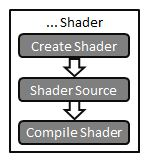Prev: OpenGL Setup Next: Creating a Program

The following figure shows the necessary steps to create a shader.The first step is creating an object which will act as a shader container. The function available for this purpose returns a handle for the container.

The syntax for this function is as follows:

[stextbox]`GLuint glCreateShader(GLenum shaderType);`

Parameter:

• `shaderType``GL_VERTEX_SHADER`, `GL_GEOMETRY_SHADER`, `GL_TESS_CONTROL_SHADER`, `GL_TESS_EVALUATION_SHADER`, or `GL_FRAGMENT_SHADER`.

Return Value:

• the shader handler

[/stextbox]

A shader program can be decomposed in many shader containers, each having some functions of the program. However, there can only be a main function for the set of each shader type in each single program.

The following step is to add some source code. The source code for a shader is a string array, although you can use a pointer to a single string.

The syntax of the function to set the source code for a shader is:

[stextbox]`void glShaderSource(GLuint shader, int numOfStrings, const char **strings, int *lengthOfStrings);`

Parameters:

• `shader` – the handler to the shader.
• `numOfStrings` – the number of strings in the array.
• `strings` – the array of strings.
• `lengthOfStrings` – an array with the length of each string, or NULL, meaning that the strings are NULL terminated.

[/stextbox]

Finally, the shader must be compiled. The function to achieve this is:

[stextbox]`void glCompileShader(GLuint shader);`

Parameters:

• `shader` – the handler to the shader.

[/stextbox]

Considering the source code is defined as a string inside the application code itself then the following snippet could be used to setup a shader:

```std::string source = "#version 330\n\
\
in vec4 Color;\n\
\
void main()\
{\
gl_FragColor = Color;\
} ";

GLuint f;
const char * ff = source.c_str();

f = glCreateShader(GL_FRAGMENT_SHADER);
glShaderSource(f, 1, &ff, NULL);
glCompileShader(f);```

Assuming the code is in a file, then the source code to achieve this could be:

```char *vs;
GLuint v;

// get a shader handler
v = glCreateShader(GL_VERTEX_SHADER);
// read the shader source from a file
vs = textFileRead(vertexFileName);
// conversions to fit the next function
const char *vv = vs;
// pass the source text to GL
glShaderSource(v, 1, &vv,NULL);
// free the memory from the source text
free(vs);
// finally compile the shader
glCompileShader(v);```

The above code uses an auxiliary function to read the text, `textFileRead`. The code for this function is now provided:

```#include <stdio.h>
#include <stdlib.h>
#include <string.h>

char *textFileRead(char *fn) {

FILE *fp;
char *content = NULL;

int count=0;

if (fn != NULL) {
fp = fopen(fn,"rt");

if (fp != NULL) {

fseek(fp, 0, SEEK_END);
count = ftell(fp);
rewind(fp);

if (count > 0) {
content = (char *)malloc(sizeof(char) * (count+1));
count = fread(content,sizeof(char),count,fp);
content[count] = '\0';
}
fclose(fp);
}
}
return content;
}```

 Prev: OpenGL Setup Next: Creating a Program

### 4 Responses to “GLSL Tutorial – Creating a Shader”

1.Remember, sizeof(char) is defined always to be 1, no matter what the platform is (the C and C++ standards both define 1 byte to be the size of a char, and sizeof to return the number of bytes needed to hold a given type). You never need to use it, unless you’re doing it for documentation purposes (and even then, it reads like, “and then multiply by the number of bytes in a byte”).

•It’s really just to be consistent with all the other mallocs, but you’r right. 🙂

2.Typo: **lenghtOfStrings** – an array with the length of each string, or NULL, meaning that the strings are NULL terminated.”

Thanks for the articles.

•Many Thanks.

This site uses Akismet to reduce spam. Learn how your comment data is processed.